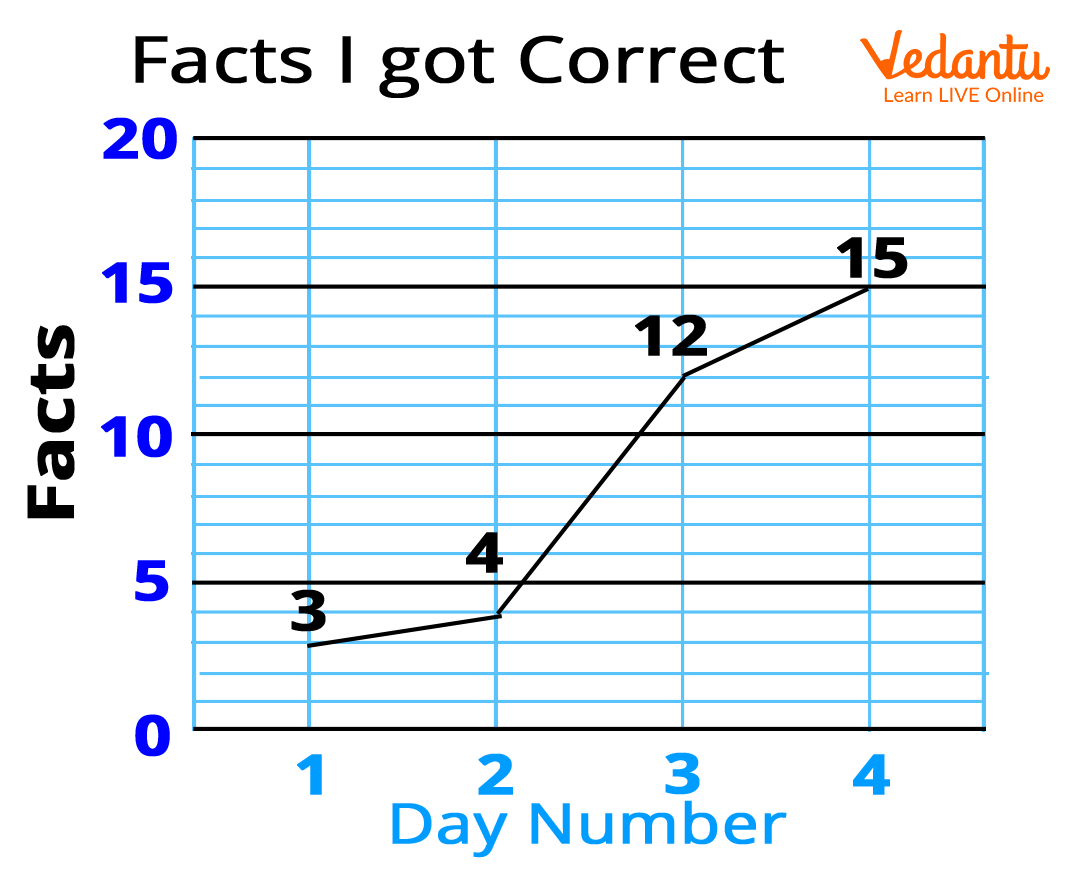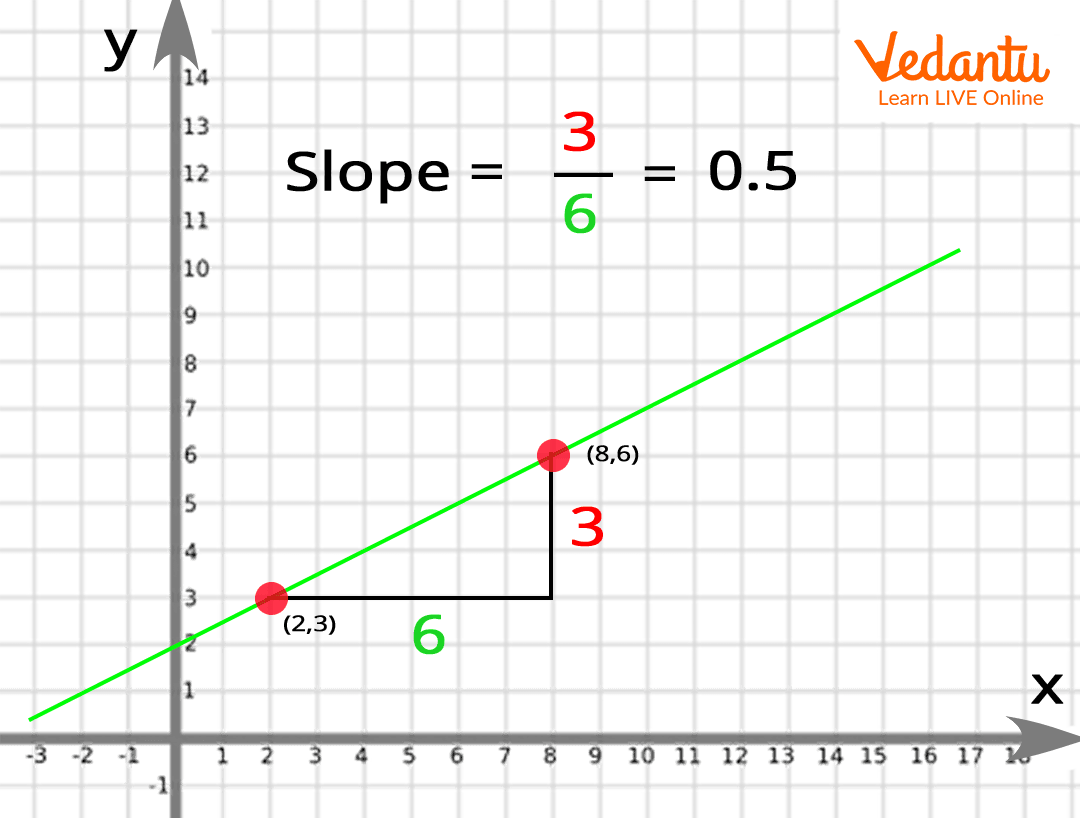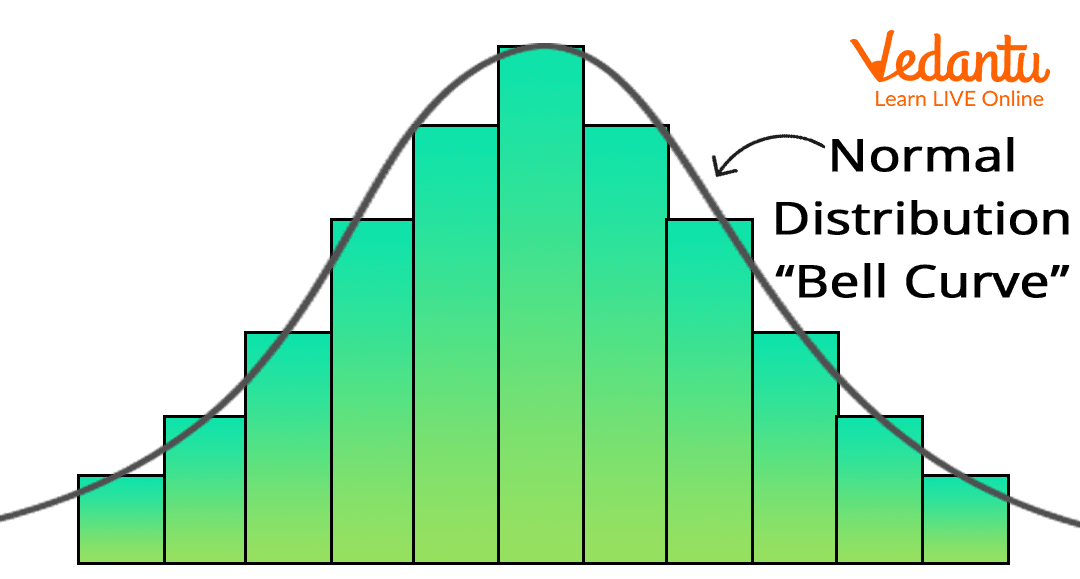Courses
Courses for Kids
Free study material
Free LIVE classes
MoreLIVE
Join Vedantu’s FREE Mastercalss

## Math Words Starting With the letter G

There are several words in mathematics that begin with the alphabet ‘g’. Thorough knowledge of these words will help kids lay a foundation for mathematics in the long run. Learning and practicing the math words that start with g will therefore enhance their mathematical vocabulary and prevent them from mixing up the meaning and concept of these words. So here’s a list of the important math words that start with the alphabet ‘g’, along with their meaning in mathematics.

• Geometry: It is a branch of mathematics that involves the theories of 2D and 3D lines, shapes, and solids. Geometry covers all the properties, theorems, and construction of these figures.

• Gallon: A gallon is a standard unit used for measuring the volume of liquids.

1 gallon = 231 cubic inches = 3.785411784 litres

• Gram: A metric unit for measuring the physical quantity, mass.

1 kilogram = 1 gram

• Geometric Mean: This is a type of average or mean, in which the quantities to be averaged are multiplied together, first. Then a square root of the product (when two quantities are given), or a cube root of the product (when three quantities are given) is calculated to obtain the final result. The value of the square root or cube root is called the geometric mean.

G.M. of a,b = ab

• Geometric Progression: It is a sequence of numbers that have a common factor, called the common ratio. Each number in the sequence is multiplied by the common ratio to obtain the next number.

An example of geometric progression: 2, 4, 8, 16, 32.

• Graph: It is a visual representation of data, displaying the relationship among variables for different constant values. It comprises a set of points and lines connecting the points. Usually, graphs are plotted in the quadrant of the x and y axes.Image of a graph

• Greatest Common Factor: It represents the number that is a common factor of two or more numbers. To calculate the greatest common factor for a set of numbers, we first need to find all the factors for the numbers. Then, the largest of the common factors of them all will give us the greatest common factor for the given set of numbers. It is also referred to as GCF.

• Gradient: The gradient of a line gives the measure of its steepness. It is also referred to as the slope of a line. For example, if a straight line is given on a graph, we will first draw a right-angled triangle taking the given straight line as the hypotenuse. The ratio of the perpendicular to the base of this right-angled triangle will give us the gradient of the given straight line.Image of the slope or gradient of a line

• Golden Ratio: The golden ratio is obtained when a line is divided into two unequal parts, such that, the ratio of the longer part to the shorter part is equal to the ratio of the whole length to the longer part.

The value of the golden ratio is 1.618033989.

Golden Ratio = 1+52

• Gross: Gross means to the total amount before any deductions. For example, gross income refers to the amount payable before deduction of income taxes and any other expenses. Another meaning of gross is 12 dozen, that is, 1 Gross = 144.

• Gaussian Distribution: It is also referred to as the Normal Distribution. When a representation of data tends to be distributed evenly around a central value, with the central value being the greatest, it is called a Normal or Gaussian distribution. The curve obtained for a Gaussian distribution is closest to the shape of a bell. Hence it is called the bell curve.Image of a histogram and the Gaussian distribution Curve

• Greater Than: The symbol for ‘greater than’ is given as >. When two numbers or quantities are compared, then the bigger number or quantity is called greater than the smaller number or quantity. For example, 6 is greater than 5, that is, 6>5.

• Giga: It is used as a prefix to many units, to represent 1 billion, that is, 10^{9}. The symbol for ‘Giga’ is G. Gigabyte, gigameter, etc. are common examples of units where this prefix is used.

## Conclusion

The above-discussed list of math words that start with g comprise some of the most commonly used mathematical terms. Learning these words will certainly equip kids with an upper hand at understanding the concepts of mathematics covered in their school curriculum. You can also refer to the lists of math words starting with common alphabets like A, B, O, V, etc. on Vedantu and guide your kids through a fun way of learning some fundamental concepts of math.

Last updated date: 03rd Oct 2023
Total views: 137.7k
Views today: 1.37k

1. What are the five most common math words starting with the letter g?

The five most common math words starting with the letter g are as follows.

• Gram

• Graph

• Geometry

• Greater Than

• Gross

2. Why should kids learn common math words?

Learning the common math words at an early age can be highly beneficial for the aptitude of kids. With prior knowledge of some of the most commonly-used math words, kids will be able to easily grasp several concepts of math taught in school. It will implant a curiosity in their minds to know about the applications of the math words. Thus, they will develop an interest in math as and when a math glossary is introduced to them through fun games or flashcards.